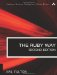# Section 5.3. Rounding Floating Point Values

### 5.2. Basic Operations on Numbers

The normal operations of addition, subtraction, multiplication, and division are implemented in Ruby much as in the typical programming language with the operators +, -, *, and /. Most of the operators are actually methods (and therefore can be overridden).

Exponentiation (raising to a power) is done with the ** operator as in older languages such as BASIC and FORTRAN. It obeys the "normal" mathematical laws of exponentiation.

`a = 64**2    # 4096 b = 64**0.5  # 8.0 c = 64**0    # 1 d = 64**-1   # 0.015625`

Division of one integer by another results in a truncated integer. This is a feature, not a bug. If you need a floating point number, make sure that at least one operand is a floating point.

`3 / 3        # 3 5 / 3        # 1 3 / 4        # 0 3.0 / 4      # 0.75 3 / 4.0      # 0.75 3.0 / 4.0    # 0.75`

If you are using variables and are in doubt about the division, Float or to_f will ensure that an operand is a floating point number.

`z = x.to_f / y z = Float(x) / y`The Ruby Way, Second Edition: Solutions and Techniques in Ruby Programming (2nd Edition)
ISBN: 0672328844
EAN: 2147483647
Year: 2004
Pages: 269
Authors: Hal Fulton

Similar book on Amazon